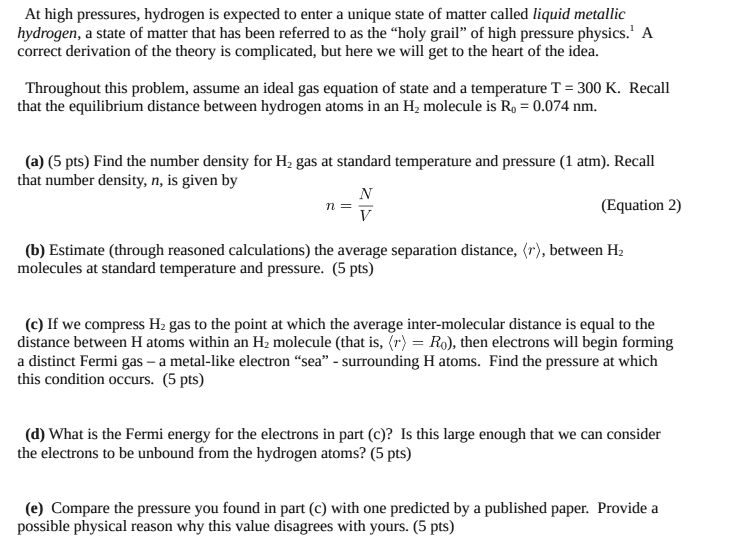# I need a help on thermodynamics physics relating to Metallic Hydrogen

• Haozi02133

#### Haozi02133

Homework Statement
If we compress H2 gas to the point at which the average inter-molecular distance is equal to the
distance between H atoms within an H2 molecule (that is,<r> = R0 ), then electrons will begin forminga distinct Fermi gas – a metal-like electron “sea” - surrounding H atoms. Find the pressure at whichthis condition occurs.
The calculated n =N/V= 2.71*10^25m^-3
<r> = 3.33nm
T=300K

I am currently stuck on part c
Relevant Equations
n = N/V = pNa/M
<r> = n^(-1/3)
R0 = 0.074nm